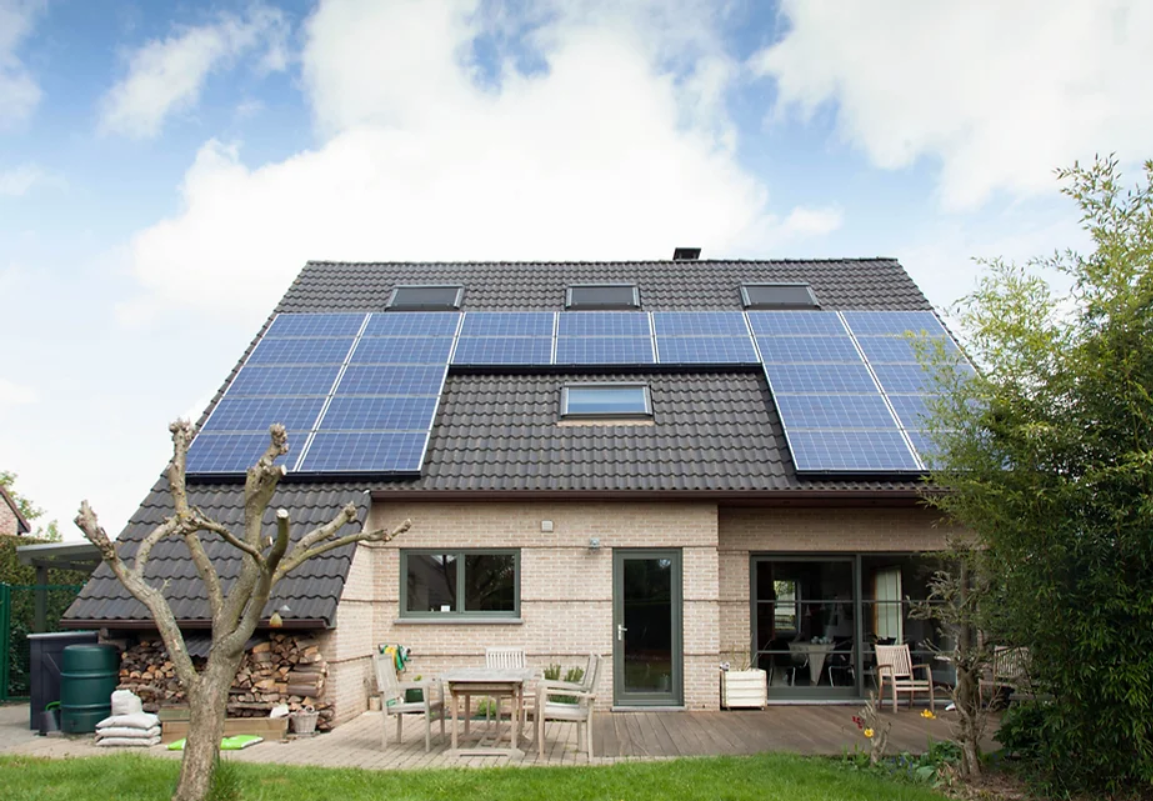Quote List

Account

## How To Calculate Solar Payback Period & ROI: Is Solar Energy Worth It?Calculating the payback period and return on investment (ROI) for a solar energy system can be a complex process, as there are many variables to consider. However, understanding these calculations can be useful for homeowners and businesses looking to invest in solar energy, as it can help them make informed decisions about whether solar is a good financial choice for them.

### How Do I Calculate the Solar Payback Period?

The payback period of a solar energy system is the amount of time it takes for the energy savings generated by the system to equal the cost of the system. In other words, it’s the point at which you’ve broken even on your investment. To calculate the payback period, you’ll need to know the following:
• The cost of the solar energy system:

This includes the cost of the panels, inverters, mounting structure, and any other necessary equipment. It’s important to get a detailed quote from a reputable solar installer to get an accurate estimate of the total cost.

• The amount of energy the system is expected to generate:

This can be calculated using tools like the Solar Calculator available on the Department of Energy’s website. You’ll need to know your location, the orientation and slope of your roof, and the size of your roof to get an accurate estimate.

• The current cost of electricity in your area:
You’ll need to know how much you currently pay for electricity in order to calculate how much you’ll save with a solar energy system. This information can be found on your monthly electricity bill.
• Any applicable incentives or subsidies:
Many states and localities offer incentives or subsidies for solar energy systems, which can significantly reduce the cost of the system. You’ll need to know what incentives are available in your area in order to calculate the true cost of the system.
Once you have this information, you can use the following formula to calculate the payback period:

### Solar Payback Formula

Payback period = Cost of solar energy system / (Annual energy savings – Annual increase in electricity costs)
For example, let’s say the cost of a solar energy system is \$20,000, it is expected to generate 10,000 kWh of electricity per year, and the current cost of electricity is \$0.10 per kWh. The annual energy savings would be \$1,000 (10,000 kWh x \$0.10/kWh), and the payback period would be 20 years (20,000 / (1,000 – 0)).

### How Do I Calculate Solar Panel Return on Investment?

The ROI of a solar energy system is a measure of how much money you can expect to make on your investment over a certain period of time. To calculate the ROI, you’ll need to know the following:
• The cost of the solar energy system:
This is the same as the cost used to calculate the payback period.
• The expected lifespan of the solar energy system:

Most solar panels have a lifespan of 25-30 years, but other components of the system may have shorter lifespans. It’s important to factor in the expected lifespan of the entire system when calculating ROI.

• The expected annual energy savings:

This is the same as the annual energy savings used to calculate the payback period.

• The expected annual increase in electricity costs:

Electricity prices are likely to increase over time, so you’ll need to estimate how much you expect your electricity costs to increase each year.

Once you have this information, you can use the following formula to calculate the ROI:

### Solar ROI Formula

ROI = (Total energy savings over the lifespan of the system – Cost of the system) / Cost of the system
For example, let’s say the cost of a solar energy system is \$20,000, it is expected to generate 10,000 kWh of electricity per year, and the current cost of electricity is \$0.10 per kWh. The expected lifespan of the system is 25 years, and you expect electricity costs to increase by 3% each year. The total energy savings over the lifespan of the system would be \$30,000 (10,000 kWh x \$0.10/kWh x 25 years), and the ROI would be 50% ((30,000 – 20,000) / 20,000).

### Conclusion: Is Solar Energy Worth It?

In order to respond to the query, “Are solar panels worth it?” In the majority of situations, yes; particularly if you can connect to the grid and minimize the financial burden of energy storage or if you have to supply power in a remote area.
It’s important to note that the payback period and ROI of a solar energy system can vary significantly depending on a variety of factors, including the size of the system, the location, and the available incentives and subsidies. It’s always a good idea to consult with a reputable solar installer to get a detailed quote and to discuss the expected payback period and ROI for your specific situation.## Don't miss out on anything

Subscribe to SolarMart Photovoltaic Solutions